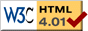# String

previous next
• Sim
• Graph
• Time Graph
• Multi Graph

Interactive simulation of a string under tension. Note that the vertical dimension is magnified to be able to see the deflection of the string.

Try dragging the string's left connection point. Try different shapes. Try changing density, tension, damping (friction), number of points, and time step. But see the section below about stability.

The math behind the simulation is shown below. Also available are: open source code, documentation and a simple-compiled version which is more customizable.

Unlike most other simulations on the myPhysicsLab website, this is based on a partial differential equation (PDE). Instead of a discrete set of variables there are an infinite number of variables corresponding to each point on the string. We approximate this in the simulation with a finite number of points which you can set.

We follow the method shown in Numerical Analysis, Sixth Edition by Richard L. Burden and J. Douglas Faires, section 12.3 Hyperbolic Partial-Differential Equations.

Note: In that edition there is an error in their Algorithm 12.4 Wave Equation Finite-Difference, where a plus sign should instead be a minus sign in step 5; compare to their equation 12.18.

## Wave Equation

See the Wikipedia article about String vibration for more information and a derivation of the wave equation.

Let

• l = length of string
• x = position along string, 0 < x < l
• t = time
• $$\alpha = \sqrt{tension/density}$$, this constant is equal to the wave speed
• u(x, t) = displacement of the string at given position and time

Here is the wave equation which governs the motion of the string:

$$\frac{\partial^2 u}{\partial t^2}(x, t) = \alpha^2 \frac{\partial^2 u}{\partial x^2}(x,t)$$

The endpoints are fixed:

$$u(0,t) = u(l,t) = 0, t > 0$$

The initial shape is given by f(x) :

$$u(x,0) = f(x), 0 \leq x \leq l$$

The initial velocity is given by g(x) :

$$\frac{\partial u}{\partial t}(x,0) = g(x), 0 \leq x \leq l$$

In the above simulation we allow choosing from a variety of initial shapes, but we assume the initial velocity is zero everywhere.

The wave equation in words says

At any given point on the string:
The acceleration of the displacement equals the curvature (2nd derivative) of the string at that point times a constant.

## Numerical Solution

Let

• m = number of points (minus one)
• h = l/m = spatial grid size
• k = time step

The numerical solution involves forming 3 arrays, each with m+1 points. These arrays represent the displacement of the string at three different times:

• previous time = now - k
• current time = now
• next time = now + k

We approximate the temporal and spatial derivatives of the wave equation from the previous and current arrays, and write new values into the "next" array. See Burden & Faires, or the source code for details.

## Stability Condition

The algorithm used here becomes unstable with certain settings for density, tension, time step k and spatial grid size h . The stability condition is:

$$\alpha k / h = \sqrt{tension/density} \; k / h \le 1$$

To keep the same stability condition:

• if you decrease spatial grid size h , you must decrease the time step k
• if you increase the tension (or decrease density), you must decrease the time step k or increase spatial grid size h
• if you increase the density (or decrease tension), you must increase the time step k or decrease spatial grid size h

This web page was first published November 2016.

previous next